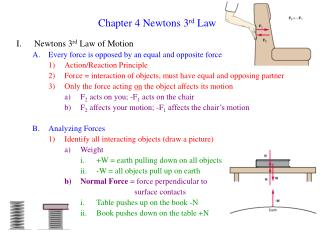DownloadDownload PresentationChapter 4 Newtons 3 rd Law

# Chapter 4 Newtons 3 rd Law

Télécharger la présentation## Chapter 4 Newtons 3 rd Law

- - - - - - - - - - - - - - - - - - - - - - - - - - - E N D - - - - - - - - - - - - - - - - - - - - - - - - - - -
##### Presentation Transcript

1. Chapter 4 Newtons 3rd Law • Newtons 3rd Law of Motion • Every force is opposed by an equal and opposite force • Action/Reaction Principle • Force = interaction of objects, must have equal and opposing partner • Only the force acting on the object affects its motion • F2 acts on you; -F1 acts on the chair • F2 affects your motion; -F1 affects the chair’s motion • Analyzing Forces • Identify all interacting objects (draw a picture) • Weight • +W = earth pulling down on all objects • -W = all objects pull up on earth • Normal Force = force perpendicular to surface contacts • Table pushes up on the book -N • Book pushes down on the table +N

2. What are the forces on the book? +W and –N +W = -N • Ftotal = maa = 0, then Ftotal = 0 = W – N = 0 • Earth has one force acting on it (-W) • Table has one force acting on it (+N) • +W and –N are not a 3rd Law pair, they just happen to be equal and opposite (that’s how the book sits still) • If +W and –N are not equal, the book would be accelerating C. How can we ever move anything? • All forces have opposite and equal partners • Only the forces acting on an object affect its motion • Cart: friction, weight, normal, mule • Mule: friction, weight, normal, cart • Mule will move if sum of forces aren’t 0 • Cart will move if sum of forces aren’t 0 Don’t affect book’s motion The only time objects don’t move is when all forces total zero!

3. Applications of Newton’s Laws • Acceleration of a car Friction (f) of the tires on the road Is opposed by –f, the road pushing the car • Moving a Chair W, N, P, f are the forces Treat vertical and horizontal separately • Skydiving • Why don’t we fall continually faster? • Air Resistance (R) is a special case of friction • Mass and Velocity determine R • Terminal Velocity = final speed due to • W = R • ~100-120 mph for a skydiver

4. Thrown Ball • P, W, R to consider • Throw: P is major force • Flies: W, R only • Connected Objects • Treat as one object: just like other examples • Treat as two objects: consider m1 and m2 and F1 and F2 FT = 36 N a = ? m1 = 10 kg F1 = ? m2 = 8 kg F2 = ?